The speed of the water in a whirlpoil varies inversely with the radius. if the water speed is 2.5 feet per second at a radius of 30 feet wha

Question

The speed of the water in a whirlpoil varies inversely with the radius. if the water speed is 2.5 feet per second at a radius of 30 feet what js the speed of the water at a radius of 3 feet?​

in progress 0
5 months 2021-08-10T20:42:33+00:00 1 Answers 39 views 0

25 feet per second.

Step-by-step explanation:

Let s represent the speed of the water and r represent the radius of the whirlpool.

The speed in a whirlpool varies inversely with the radius. Therefore, we can write that: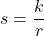Where k is the constant of variation.

We know that the speed is 2.5 feet per second when the radius is 30 feet.

So, s = 2.5 when r = 30. Substitute: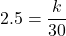Solve for k: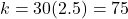So, our equation is: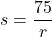Then when the radius is three feet, r = 3. Evaluate for s: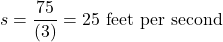So, the speed of the water in a whirlpool with a radius of three feet is 25 feet per second.